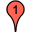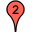Locate weird geometrical shapes on the places you love

Tool created by Mariano Tomatis

The tool here provided can be used to find weird (maybe sacred?) geometries on maps provided by Google®, starting from two points defined by the user.

1) Specify in decimal format latitudes and longitudes of the two key points of your geometry (use a DMS-to-decimal converter if you need it).

You can verify the correctness of your coords by clicking "View" buttons.

2) Select one of the four shapes from the icons on the left and click on it, to draw it on the map starting from the two points.° Latitude View » ° Longitude° Latitude View » ° Longitude

How to get latitude and longitude from Google Maps

Center the map on the point you want and paste in the URL bar of your browser this command:

javascript:void(prompt('',gApplication.getMap().getCenter()));

Default settings

Default settings refer to the Magdala Tower in Rennes-le-Château and the Blanchefort Castle, both in the French region of Aude. According to Henry Lincoln, the mountain top of Rennes-le-Château and of four other adjacent mountain tops precisely mark out a pentagram, the tips of a five-pointed star that stretches across the landscape.

Coordinates

Starting from the two points specified by the user, the calculation of vertices is performed with trigonometrical formulas, with a proper correction because of the spherical surface of the Earth.

At first, the longitude of each point is divided by cosine of latitude. Then, the coordinates of other points are calculated. At the end, all points are multiplied by cosine of latitude.

Triangles

Two points on a map define two distinct equilateral triangles.

Given the coordinates of the two points (ll1,lt1) and (ll2,lt2), points #3 are defined this way:

 Triangle A Point #A3 ll3 = cos(120°)×(ll2-ll1) - sin(120°)×(lt2-lt1) + ll2 lt3 = sin(120°)×(ll2-ll1) + cos(120°)×(lt2-lt1) + lt2
 Triangle B Point #B3 ll3 = cos(120°)×(ll1-ll2) - sin(120°)×(lt1-lt2) + ll1 lt3 = sin(120°)×(ll1-ll2) + cos(120°)×(lt1-lt2) + lt1

Squares

Two points on a map define three distinct squares (they may define a side in two different ways A,B or its diagonal in one way C).

For triangle C, it is useful to define the median point (llM,ltM) as ((ll1+ll2)/2,(lt1+lt2)/2).

 Square A Point #A3 ll3 = cos(90°)×(ll2-ll1) - sin(90°)×(lt2-lt1) + ll2 lt3 = sin(90°)×(ll2-ll1) + cos(90°)×(lt2-lt1) + lt2 Point #A4 ll4 = cos(90°)×(ll3-ll2) - sin(90°)×(lt3-lt2) + ll3 lt4 = sin(90°)×(ll3-ll2) + cos(90°)×(lt3-lt2) + lt3
 Square B Point #B3 ll3 = cos(90°)×(ll1-ll2) - sin(90°)×(lt1-lt2) + ll1 lt3 = sin(90°)×(ll1-ll2) + cos(90°)×(lt1-lt2) + lt1 Point #B4 ll4 = cos(90°)×(ll3-ll1) - sin(90°)×(lt3-lt1) + ll3 lt4 = sin(90°)×(ll3-ll1) + cos(90°)×(lt3-lt1) + lt3
 Square C Point #C3 ll3 = cos(90°)×(llM-ll1) - sin(90°)×(ltM-lt1) + llM lt3 = sin(90°)×(llM-ll1) + cos(90°)×(ltM-lt1) + ltM Point #C4 ll4 = cos(90°)×(llM-ll2) - sin(90°)×(ltM-lt2) + llM lt4 = sin(90°)×(llM-ll2) + cos(90°)×(ltM-lt2) + ltM

Pentacles

Two points on a map define four distinct pentacles.

 Pentacle A Point #A3 ll3 = cos(72°)×(ll2-ll1) - sin(72°)×(lt2-lt1) + ll2 lt3 = sin(72°)×(ll2-ll1) + cos(72°)×(lt2-lt1) + lt2 Point #A4 ll4 = cos(72°)×(ll3-ll2) - sin(72°)×(lt3-lt2) + ll3 lt4 = sin(72°)×(ll3-ll2) + cos(72°)×(lt3-lt2) + lt3 Point #A5 ll5 = cos(72°)×(ll4-ll3) - sin(72°)×(lt4-lt3) + ll4 lt5 = sin(72°)×(ll4-ll3) + cos(72°)×(lt4-lt3) + lt4
 Pentacle B Point #B3 ll3 = cos(72°)×(ll1-ll2) - sin(72°)×(lt1-lt2) + ll1 lt3 = sin(72°)×(ll1-ll2) + cos(72°)×(lt1-lt2) + lt1 Point #B4 ll4 = cos(72°)×(ll3-ll1) - sin(72°)×(lt3-lt1) + ll3 lt4 = sin(72°)×(ll3-ll1) + cos(72°)×(lt3-lt1) + lt3 Point #B5 ll5 = cos(72°)×(ll4-ll3) - sin(72°)×(lt4-lt3) + ll4 lt5 = sin(72°)×(ll4-ll3) + cos(72°)×(lt4-lt3) + lt4
 Pentacle C Point #C3 ll3 = cos(144°)×(ll2-ll1) - sin(144°)×(lt2-lt1) + ll2 lt3 = sin(144°)×(ll2-ll1) + cos(144°)×(lt2-lt1) + lt2 Point #C4 ll4 = cos(144°)×(ll3-ll2) - sin(144°)×(lt3-lt2) + ll3 lt4 = sin(144°)×(ll3-ll2) + cos(144°)×(lt3-lt2) + lt3 Point #C5 ll5 = cos(144°)×(ll4-ll3) - sin(144°)×(lt4-lt3) + ll4 lt5 = sin(144°)×(ll4-ll3) + cos(144°)×(lt4-lt3) + lt4
 Pentacle D Point #D3 ll3 = cos(144°)×(ll1-ll2) - sin(144°)×(lt1-lt2) + ll1 lt3 = sin(144°)×(ll1-ll2) + cos(144°)×(lt1-lt2) + lt1 Point #D4 ll4 = cos(144°)×(ll3-ll1) - sin(144°)×(lt3-lt1) + ll3 lt4 = sin(144°)×(ll3-ll1) + cos(144°)×(lt3-lt1) + lt3 Point #D5 ll5 = cos(144°)×(ll4-ll3) - sin(144°)×(lt4-lt3) + ll4 lt5 = sin(144°)×(ll4-ll3) + cos(144°)×(lt4-lt3) + lt4

© 2019 Mariano Tomatis Antoniono • Dharma Initiative • Mathematical Forecasting Initiative • Italian Division (Turin)